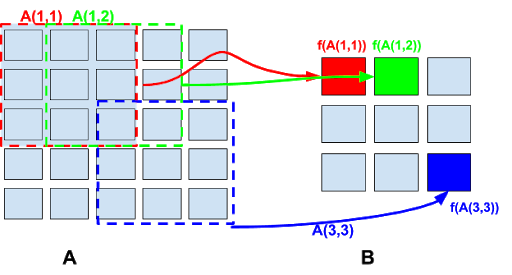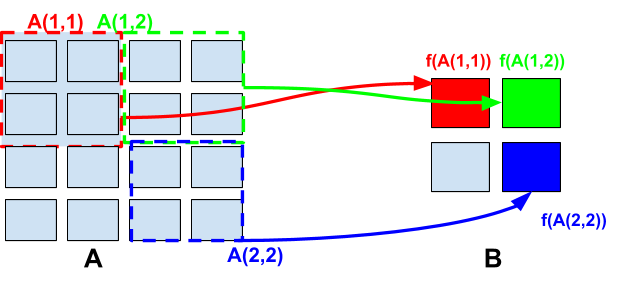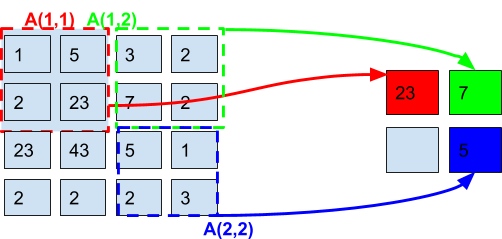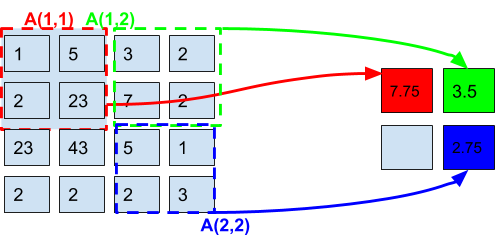•written on Jan 06, 2020
by Martin Rupp

### Artificial Intelligence - Understanding the Main Operations in Convolution Neural Networks for RADAR Data Classification

ATC-Training Artificial Intelligence Theory & Research

#RADAR data classification mainly relies on convolutional neural networks. In this article, we shall detail and explain the main operations performed by Convolution networks in order to classify RADAR data.

First of all, we need to define what is the convolution operation, which is at the root of such networks. This article is using a lot of maths. If you are looking at it from a skill development point of view, just try to get a bit of the general meaning. The SkyRadar training equipment will help to get qualified on machine learning and artificial intelligence in a hands-on approach.

If you are an engineer, a university professor or a radar expert who wants to apply this technology, take your time to go through it. SkyRadar's hands-on training equipment may help you to rapidly enter the world of AI. To get the whole picture, you may look at the complete series of articles or at our white paper on Artificial Intelligence.

# Convolution Operation

The Convolution operation is similar to the product between two matrices.

If M and N are two matrices then (MN)ij = sum_(k)MikNkj

The convolution operation between two matrices is more complicated.

A matrix K, usually “small” plays the role of a "filter” to regularize an other matrix, A, using the formula :

(A * K)ij = sum_(s,t)KstAi-s, j-t

In this formula, s and t run through, respectively -a to +a and -b to +b which implies that the filter matrix has the dimensions 2a+1 x 2b+1.

Usually 2a+1 = 2b+1 = F.

The size of the convoluted matrix is given by C = ((L-F+2P)/S)+1.

L is the size of the input matrix A , F the size of the filter matrix , S the stride, and P the eventual padding which is applied to the input matrix A.

In the following, we illustrate the geometrical meaning of the convolution operation over two matrices:

In the above representation, L = 5, F = 3, S = 1, P = 0 and therefore C = (5-3)/1+1=3.

The convolution operation is well known in image processing.

Convolution alone is not enough, reducing the amount of samples is often needed after a convolution operation. This is usually done through pooling.

# Pooling

Pooling is a practice of subsampling. A “pool” of the input matrix is being processed and converted into a single value filling a new entry on the output matrix.

When pooling a matrix, several arguments must be considered:

• The stride. This is the translation value which can be vertical or horizontal. E.g it represents how many cells the pool will “shift” in a direction.
• The filter length and width.. This is the pool dimension.The pool is a square submatrix of the input matrix of constant dimensions.

Let as take the following example:

A is the input matrix. It is a square matrix of dimension n.

If the stride is S and if the pool has width and length m, then the output matrix, e.g., the “pooled” matrix is B and such that:

Bij = f(Akl, S x i <= k < S x i + m, S x j  <= l < S x j + m )

Where f is a numerical function with m2 arguments.

If we note A(i,j) the pooling submatrix shifted horizontally by i strides and vertically by j strides, then we can write:

Bij = F(A(i,j))

The dimensions of the pooled matrix - say - N x N are such that S.(N-1)+m = n.

Or N = (n-m)/S+1. In the case of S = m = 1 we have N = n and the pooling operation do not perform any subsampling.

Here we represent the result of pooling operations with different values of S.

The following image shows a pooling operation on an input 5x5 matrix A , with a stride of 1 and a pool submatrix of dimensions 3x3:Here we have 3x3 submatrixes A(i,j) and the output matrix has dimension NxN
where N = (5-3)/1+1=3

Next we represent a pooling operation on a 4x4 input matrix with a stride of 2 and a pooling submatrix of dimensions 2x2.The output matrix has dimension NxN where N = (4-2)/2+1 = 2.

## Max-Pooling

Max-pooling is simply the operation of pooling with f= Max

This mean that Bij = Max(A(i,j))## Average-Pooling

Max-pooling is the operation of pooling with  f= Mean . The mean function can be for example an arithmetical mean.

This mean that Bij = Mean(A(i,j))# Softmax

The softmax operation is a normalizing operation. It takes as input a vector, say,
a = (a1, ..., an) and outputs a new vector of identical dimension, sigma(a) given by the following formula:

sigma(a)=(e^(a_1)/ (sum_(i=1)^n e^(a_i)) , ..., e^(a_i)/[ sum_(i=1)^n e^(a_i)) , ..., e^(a_n)/[ sum_(i=1)^n e^(a_i)) )

Softmax is used for multi-class classification operations, in the final layer of the convolution network.

In a next article, we will build an anatomy of a convolutional network and explain why these operations are used for classification of RADAR data.